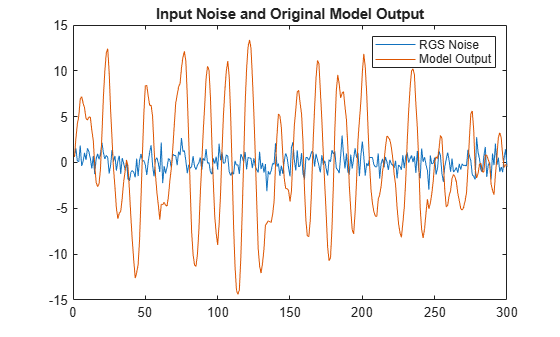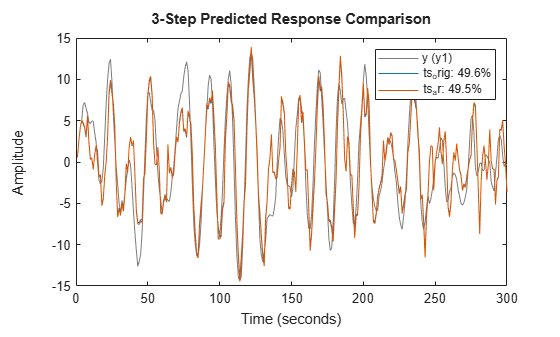Identify Time Series Models at the Command Line

This example shows how to simulate a time series and use parametric and nonparametric methods to estimate and compare time series models.

Generate Model and Simulate Model Output

Generate time series data by creating and simulating an autoregressive (AR) polynomial model ts_orig of the form ${\mathit{y}}_{\mathit{k}}={\mathit{a}}_{1}\text{\hspace{0.17em}}{\mathit{y}}_{\mathit{k}-1}+{\mathit{a}}_{2}{\text{\hspace{0.17em}}\mathit{y}}_{\mathit{k}-2}+{\mathit{e}}_{\mathit{k}}$, where ${\mathit{e}}_{\mathit{k}}$ is random Gaussian noise. This noise represents an unmeasured input to the model. Since the model is a time series, there are no measured inputs.

Before calculating ${\mathit{e}}_{\mathit{k}}$, initialize the random number generator seed so that the noise values are repeatable.

ts_orig = idpoly([1 -1.75 0.9]);
rng('default')
e = idinput(300,'rgs');

Simulate the observed output y_obs of this model and convert y_obs to an iddata object y with the default sample time of one second. Plot the model output together with the input noise.

y_obs = sim(ts_orig,e);
y = iddata(y_obs);

plot(e)
hold on
plot(y_obs)
title('Input Noise and Original Model Output')
legend('RGS Noise','Model Output')
hold offEstimate Model and Compare Spectra

The functions etfe and spa provide two nonparametric techniques for performing spectral analysis. Compare the estimated power spectra from etfe and spa to the original model.

ts_etfe = etfe(y);
ts_spa = spa(y);
spectrum(ts_etfe,ts_spa,ts_orig);
legend('ts_{etfe}','ts_{spa}','ts_{orig}')Now estimate a parametric model using the AR structure. Estimate a second-order AR model and compare its spectrum with the original model and the spa estimate.

ts_ar = ar(y,2);
spectrum(ts_spa,ts_ar,ts_orig);
legend('ts_{spa}', 'ts_{ar}', 'ts_{orig}')The AR model spectrum fits the original model spectrum more closely than the nonparametric models.

Estimate and Compare Covariance

Calculate the covariance function for the original model and the AR model by convolving each model output with itself.

ir_orig = sim(ts_orig,[1;zeros(24,1)]);
Ry_orig = conv(ir_orig,ir_orig(25:-1:1));
ir_ar = sim(ts_ar,[1;zeros(24,1)]);
Ry_ar = conv(ir_ar,ir_ar(25:-1:1));

Also estimate the covariance Ry directly from the observed outputs y using xcorr.

Ry = xcorr(y.y,24,'biased');

Plot and compare the original and estimated covariances.

plot(-24:24'*ones(1,3),[Ry_orig,Ry_ar,Ry]);
legend('Ry_{orig}', 'Ry_{ar}', 'Ry')The covariance of the estimated AR model, Ry_ar, is closer to the original covariance Ry_orig.

Predict and Compare Model Outputs

Compare the three-step prediction accuracy, or fit percentage, for the original model and the AR model, using the function compare. Here, compare computes the predicted response of the ts_orig and ts_ar models with the original model output data y, assuming unmeasured input ${\mathit{e}}_{\mathit{k}}$ is zero. The fourth argument, 3, is the number of steps to predict.

compare(y,ts_orig,ts_ar,3);The percentages in the legend are the fit percentages, which represent goodness of fit. The prediction accuracy is far from 100% even for the original model because the unmeasured model input ${\mathit{e}}_{\mathit{k}}$ is not accounted for in the prediction process. The fit value for the estimated AR model is close to the original model, indicating that the AR model is a good estimate.